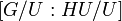# Index of a closed subgroup in a profinite group

Suppose$G$ is a profinite group and$H$ is a closed subgroup of$G$. The index of$H$ in$G$, in the profinite group sense, is defined as the following supernatural number:
1. It is the lcm (in the supernatural number sense) of the values of the index$[G/U:HU/U]$ where$U$ varies over the open normal subgroups of$G$. Note that if$U$ is an open normal subgroup of the profinite group$G$, then$G/U$ is finite, so the index is actually a finite number.
2. It is the lcm (in the supernatural number sense) of the values of the index$[G:V]$ where$V$ ranges over the open normal subgroups of$G$ containing$H$.